# B&O Tech: The Cube

#51 in a series of articles about the technology behind Bang & Olufsen loudspeakers

Sometimes, we have journalists visiting Bang & Olufsen in Struer to see our facilities. Of course, any visit to Struer means a visit to The Cube – our room where we do almost all of the measurements of the acoustical behaviour of our loudspeaker prototypes. Different people ask different questions about that room – but there are two that come up again and again:

• Why is the room so big?
• Why isn’t it an anechoic chamber?

Of course, the level of detail of the answer is different for different groups of people (technical journalists from audio magazines get a different level of answer than lifestyle journalists from interior design magazines). In this article, I’ll give an even more thorough answer than the one the geeks get. :-)

## Introduction #1 – What do we want?

Our goal, when we measure a loudspeaker, is to find out something about its behaviour in the absence of a room. If we measured the loudspeaker in a “real” room, the measurement would be “infected” by the characteristics of the room itself. Since everyone’s room is different, we need to remove that part of the equation and try to measure how the loudspeaker would behave without any walls, ceiling or floor to disturb it.

So, this means (conceptually, at least) that we want to measure the loudspeaker when it’s floating in space.

## Introduction #2 – What kind of measurements do we do?

Basically, the measurements that we perform on a loudspeaker can be boiled down into four types:

1. on-axis frequency response
2. off-axis frequency responses
3. directivity
4. power response

Luckily, if you’re just a wee bit clever (and we think that we are…), all four of these measurements can be done using the same basic underlying technique.

The very basic idea of doing any audio measurement is that you have some thing whose characteristics you’re trying to measure – the problem is that this thing is usually a link in a chain of things – but you’re only really interested in that one link. In our case, the things in the audio chain are electrical (like the DAC, microphone preamplifier, and ADC) and acoustical (like the measurement microphone and the room itself).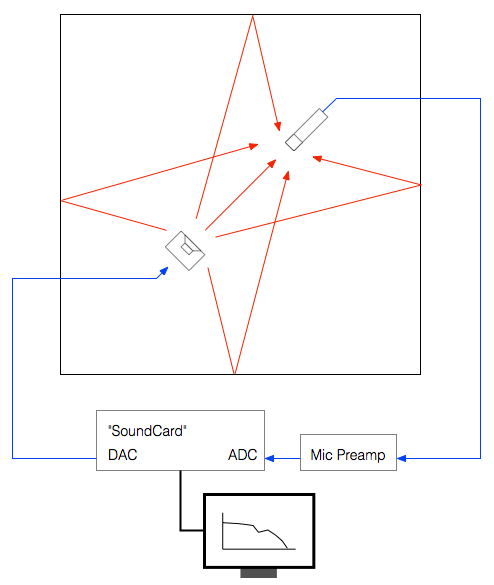Figure 2: A block diagram of the measurement system in The Cube. The acoustical signals in the room are shown in red. Analogue electrical connections around shown in blue. (That thing on the bottom is supposed to look like a computer showing a magnitude response plot…)

The computer sends a special signal (we’ll come back to that…) out of its “sound card” to the input of the loudspeaker. The sound comes out of the loudspeaker and comes into the microphone (however, so do all the reflections from the walls, ceiling and floor of the Cube). The output of the microphone gets amplified by a preamplifier and sent back into the computer. The computer then “looks at” the difference between the signal that it sent out and the signal that came back in. Since we already know the characteristics of the sound card, the microphone and the mic preamp, then the only thing remaining that caused the output and input signals to be different is the loudspeaker.

## Introduction #3 – The signal

There are lots of different ways to measure an audio device. One particularly useful way is to analyse how it behaves when you send it a signal called an “impulse” – a click. The nice thing about a theoretically perfect click is that it contains all frequencies at the same amplitude and with a known phase relationship. If you send the impulse through a device that has an “imperfect” frequency response, then the click changes its shape. By doing some analysis using some 200-year old mathematical tricks (called “Fourier analysis“), we can convert the shape of the impulse into a plot of the magnitude and phase responses of the device.

So, we measure the way the device (in our case, a loudspeaker) responds to an impulse – in other words, its “impulse response”.

There are three things to initially notice in this figure.

• The first is the time before the first impulse comes in. This is the time it takes the sound to travel from the loudspeaker to the microphone. Since we normally make measurements at a distance of 3 m, this is about 8.7 ms.
• The second is the fact that the impulse doesn’t look perfect. That’s because it isn’t – the loudspeaker has made it different.
• The third is the presence of the wall reflection. (In real life, we see 6 of these – 4 walls, a ceiling and the floor – but this example is a simulation that I created, just to show the concept.)

In order to get a measurement of the loudspeaker in the absence of a room, we have to get rid of those reflections… In this case, all we have to do is to tell the computer to “stop listening” before that reflection arrives. The result is the impulse response of the loudspeaker in the absence of any reflections – which is exactly what we want.

• We make this measurement “on-axis” (usually directly in front of the loudspeaker, at some specific height which may or may not be the same height as the tweeter) to get a basic idea to begin with.
• We can then rotate the crane (the device hanging from the ceiling that the loudspeaker is resting on) with a precision of 1º. This allows us to make an off-axis measurement at any horizontal angle.
• In order to find the loudspeaker’s directivity (like Figure 5, 6, and 7 in this posting), we start by making a series of measurements (typically, every 5º for all 360º around the loudspeaker). We then tell the computer to compare the difference between each off-axis measurement and the on-axis measurement (because we’re only interested in how the sound changes with rotation – not its actual response at a given off-axis angle – we already got that in the previous measurement.
• Finally, we have to get the power response. This one is a little more tricky. The power response is the behaviour of the loudspeaker when measured in all directions simultaneously. Think of this as putting the loudspeaker in the centre of a sphere with a diameter of 3 m made of microphones. We send a signal out of the loudspeaker and measure the response at all points on the sphere, add them all together and see what the total is. This is an expensive way to do this measurement, since microphones cost a lot… An easier way is to use one microphone and rotate the loudspeaker (both horizontally and vertically) and do a LOT of off-axis measurements – not just rotating around the loudspeaker,  but also going above and below it. We do each of these measurements individually, and then add the results to get a 3D sum of all responses in all directions. That total is the power response of the loudspeaker – a measurement of the total energy the loudspeaker radiates into the room.

## The original questions…

Great. That’s a list of the basic measurements that come out of The Cube. However, I still have’t directly answered the original questions…

Let’s take the second question first: “Why isn’t The Cube an anechoic chamber?”

This raises the question: “What’s an anechoic chamber?” An anechoic chamber is a room whose walls are designed to be absorptive (typically to sound waves, although there are some chambers that are designed to absorb radio waves – these are for testing antennae instead of loudspeakers). If the walls are perfectly absorptive, then there are no reflections, and the loudspeaker behaves as if there are no walls.

So, this question has already been answered – albeit indirectly. We do an impulse response measurement of the loudspeaker, which is converted to magnitude and phase response measurements. As we saw in Figure 5, the reflections off the walls are easily visible in the impulse response. Since, after the impulse response measurement is done, we can “delete” the reflection (using a process called “windowing”) we end up with an impulse response that has no reflections. This is why we typically say that The Cube is “pseudo-anechoic” – the room is not anechoic, but we can modify the measurements after they’re done to be the same as if it were.

Now to the harder question to answer: “Why is the room so big?”

Let’s say that you have a device (for example, a loudspeaker), and it’s your job to measure its magnitude response. One typical way to do this is to measure its impulse response and to do a DFT (or FFT) on that to arrive at a magnitude response.

Let’s also say that you didn’t do your impulse response measurement in a REAL free field (a space where there are no reflections – the wave is free to keep going forever without hitting anything) – but, instead, that you did your measurement in a real space where there are some reflections. “No problem,” you say “I’ll just window out the reflections” (translation: “I’ll just cut the length of the impulse response so that I slice off the end before the first reflection shows up.”)

This is a common method of making a “pseudo-anechoic” measurement of a loudspeaker. You do the measurement in a space, and then slice off the end of the impulse response before you do an FFT to get a magnitude response.

Generally speaking, this procedure works fairly well… One thing that you have to worry about is a well-known relationship between the length of the impulse response (after you’ve sliced it) and the reliability of your measurement. The shorter the impulse response, the less you can trust the low-frequency result from your FFT. One reason for this is that, when you do an FFT, it uses a “slice” of time to convert the signal into a frequency response. In order to be able to measure a given frequency accurately, the FFT math needs at least one full cycle within the slice of time. Take a look at Figure 6, below.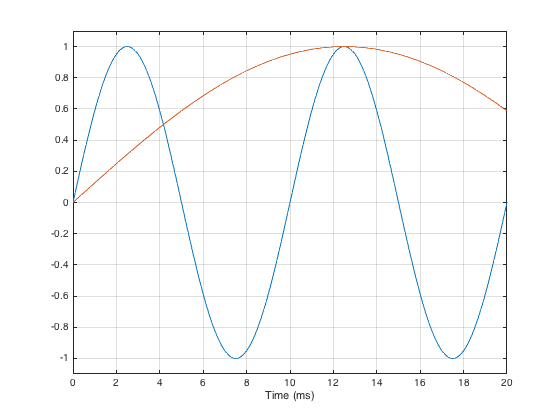Figure 6: A 100 Hz (blue) and a 20 Hz (red) sine tone, plotted for the first 20 ms.

As you can see in that plot, if the slice of time that we’re looking at is 20 ms long, there is enough time to “see” two complete cycles of a 100 Hz sine tone (in blue). However, 20 ms is not long enough to see even one half of a cycle of a 20 Hz sine tone (in red).

However, there is something else to worry about – a less-well-known relationship between the level and extension of the low-frequency content of the device under test and the impulse response length. (Actually, these two issues are basically the same thing – we’re just playing with how low is “low”…)

Let’s start be inventing a loudspeaker that has a perfectly flat on-axis magnitude response but a low-frequency limitation with a roll-off at 10 Hz. I’ve simulated this very unrealistic loudspeaker by building a signal processing flow as shown in Figure 7.

If we were to do an impulse response measurement of that system, it would look like the plot in Figure 8, below.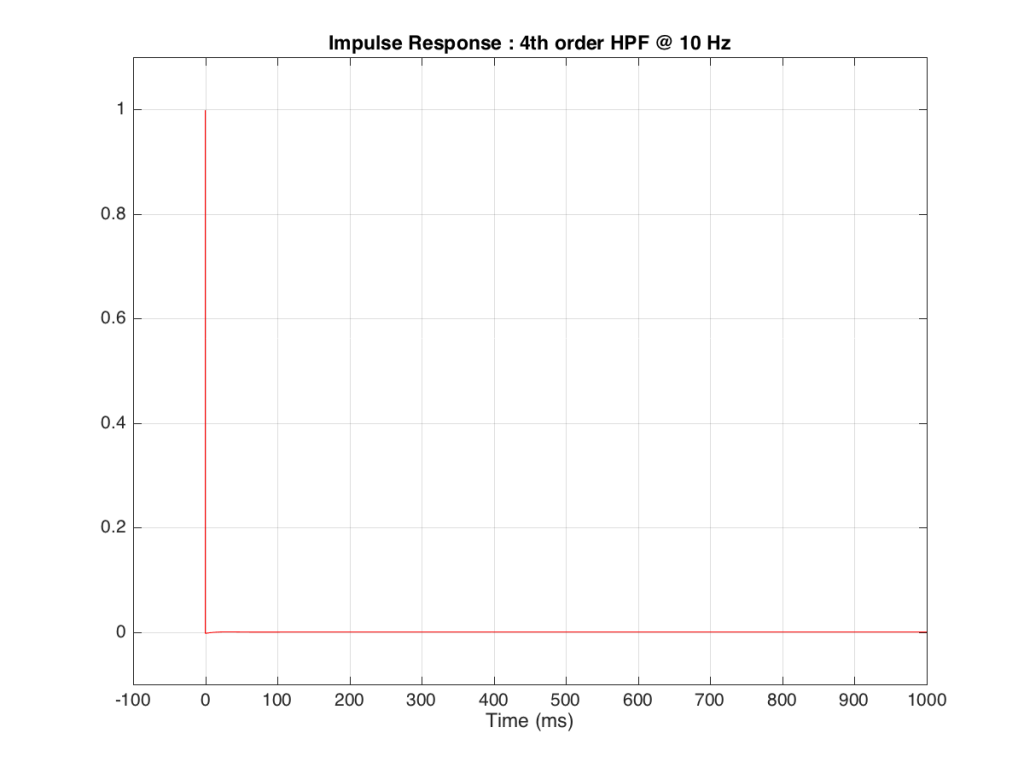Figure 8: As you can see, this is almost a perfect impulse. It goes to a value of 1 at time 0 (therefore there’s no delay) and then it’s a value of 0 for the rest of all time… Or is it? Look very carefully at the line just after the impulse. Notice how it dips just below the 0 line? Let’s zoom in on that…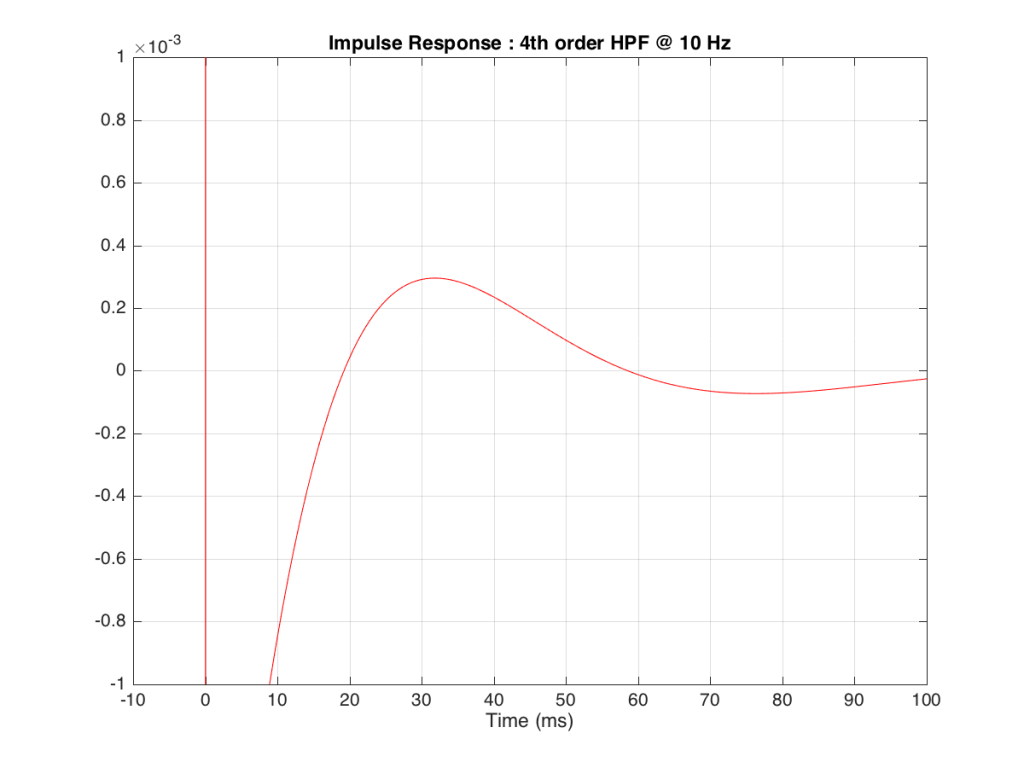Figure 9: Zooming in on Figure 8.

Figure 9, above shows a closeup of what happens just after the impulse. Notice that the signal drops below 0, then swings back up, then negative again. In fact, this keeps happening – the signal goes positive, negative, positive, negative – theoretically for an infinite amount of time – it never stops. (This is why the filters that I used to make this high pass are called “IIR” filters or “Infinite Impulse Response” filters.)

The problem is that this “ringing” in time (to infinity) is very small. However, it’s more easily visible if we plot it on a logarithmic scale, as shown below in Figure 10.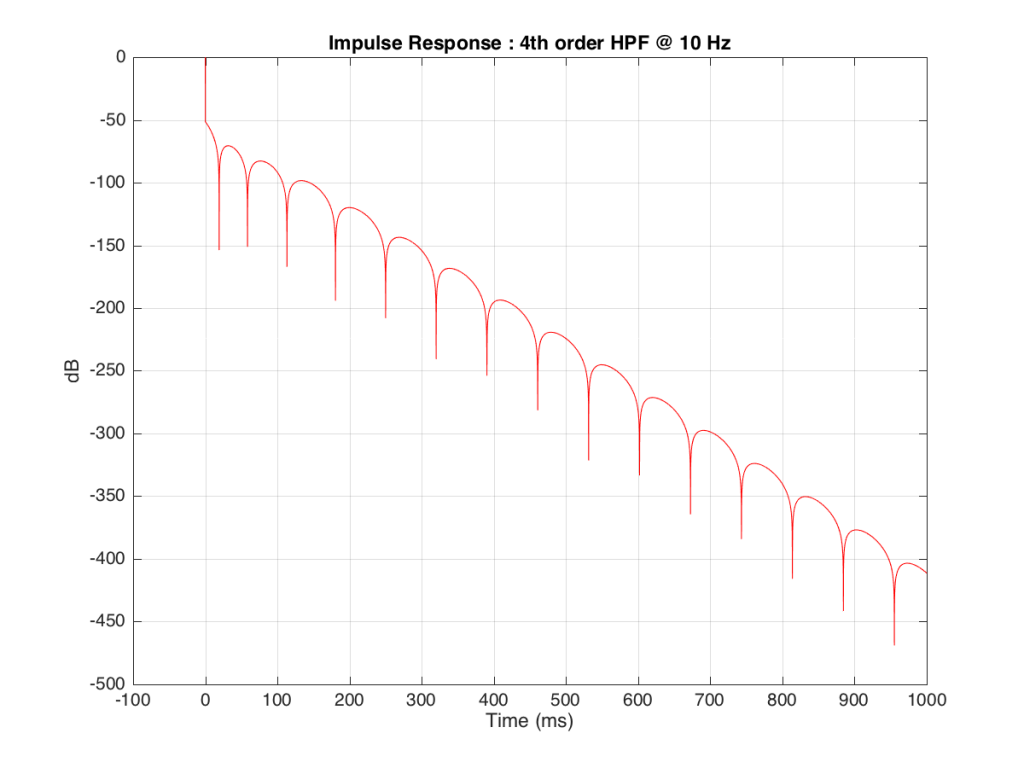Figure 10: The same plot as shown in Figure 8, plotted on a logarithmic scale by converting to decibels.

As you can see there, after 1 second (1000 ms) the oscillation caused by the filtering has dropped by about 400 dB relative to the initial impulse (that means it has a level of about 0.000 000 000 000 000 000 01 if the initial impulse has a value of 1). This is very small – but it exists. This means that, if we “cut off” the impulse to measure its frequency response, we’ll be cutting off some of the signal (the oscillation) and therefore getting some error in the conversion to frequency. The question then is: how much error is generated when we shorten the impulse length?

We won’t do an analysis of how to answer this question – I’ll just give some examples. Let’s take the total impulse response shown in Figure 6 and cut it to different lengths – 10, 15, 20, 25, 30 and 1000 ms. For each of those versions, I’ll take an FFT and look at the resulting magnitude response. These are shown below in Figure 11.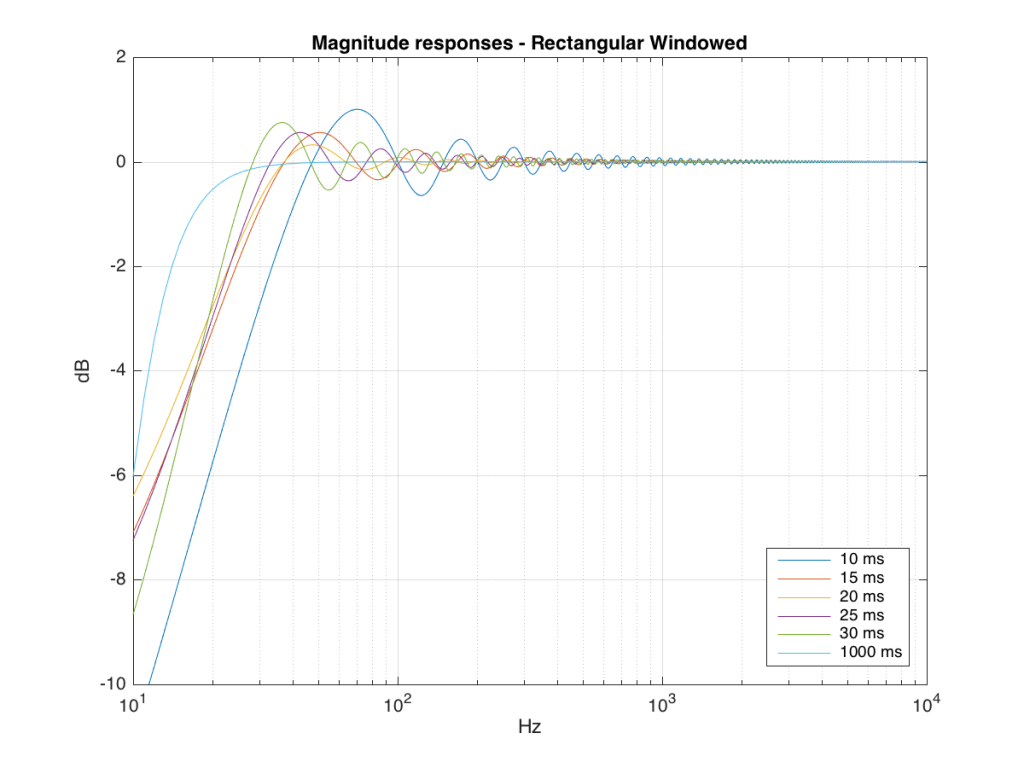Figure 11: The magnitude responses resulting from taking an FFT of a shortened portion of a single impulse response plotted in Figure 8.

We’ll assume that the light blue curve in Figure 9 is the “reference” since, although it has some error due to the fact that the impulse response is “only” one second long, that error is very small. You can see in the dark blue curve that, by doing an FFT on only the first 10 ms of the total impulse response, we get a strange behaviour in the result. The first is that we’ve lost a lot in the low frequency region (notice that the dark blue curve is below the light blue curve at 10 Hz). We also see a strange bump at about 70 Hz – which is the beginning of a “ripple” in the magnitude response that goes all the way up into the high frequency region.

The amount of error that we get – and the specific details of how wrong it is – are dependent on the length of the portion of the impulse response that we use.

If we plot this as an error – how wrong is each of the curves relative to our reference, the result looks like Figure 12.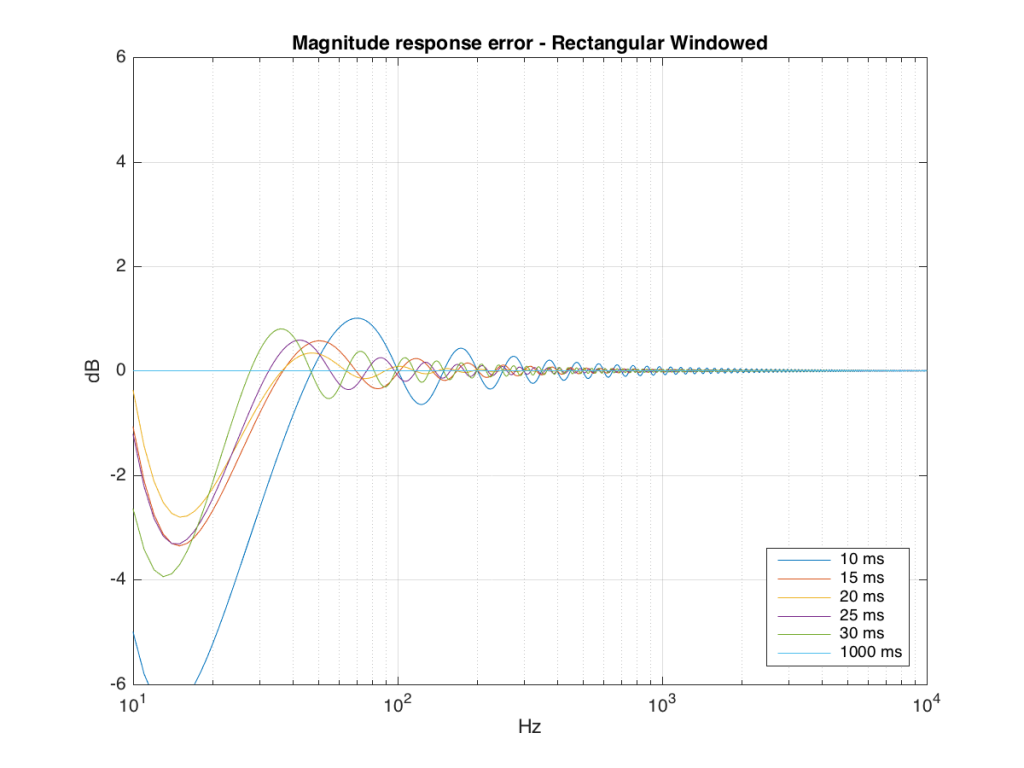Figure 12: The magnitude of the error resulting from using too-short an impulse response to calculate the magnitude response. This is the “reference” curve in Figure 9 subtracted from each curve in the same plot.

## So what?

As you can see there, using a shorted impulse response produces an error in our measurement when the signal has a significant low frequency output. However, as we said above, we shorten the impulse response to delete the early reflections from the walls of The Cube in our measurement to make it “pseudo-anechoic”. This means, therefore, that we must have some error in our measurement. In fact, this is true – we do have some error in our measurement – but the error is smaller than it would have been if the room had been smaller. A bigger room means that we can have a longer impulse response which, in turn, means that we have a more accurate magnitude response measurement.

“So why not use an anechoic chamber and not mess around with this ‘pseudo-anechoic’ stuff?” I hear you cry… This is a good idea, in theory – however, in practice, the problem that we see above is caused by the fact that the loudspeaker has a low-frequency output. Making a room anechoic at a very low frequency (say, 10 Hz) would be very expensive AND it would have to be VERY big (because the absorptive wedges on the walls would have to be VERY deep – a good rule of thumb is that the wedges should be 1/4 of the wavelength of the lowest frequency you’re trying to absorb, and a 10 Hz wave has a wavelength of 34.4 m, so you’d need wedges about 8.6 m deep on all surfaces… This would therefore be a very big room…)

## Appendix 1 – Tricks

Of course, there are some tricks that can be played to make the room seem bigger than it is. One trick that we use is to do our low-frequency measurements in the “near field” which is much closer than 3 m from the loudspeaker, as is shown in Figure 13 below. The advantage of doing this is that it makes the direct sound MUCH louder than the wall reflections (in addition to making the difference in their time of arrival at the microphone slightly longer) which reduces their impact on the measurement. The problem with doing near-field measurements is that you are very sensitive to distance – and you typically have to assume that the loudspeaker is radiating omnidirectional – but this is a fairly safe assumption in most cases.